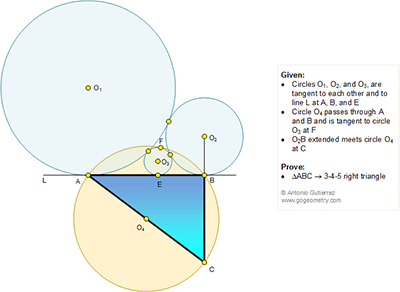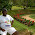## Monday, July 4, 2016

### Geometry Problem 1229: Three Tangent Circles, Midpoint, Common Tangent Line, 3-4-5 Right Triangle

Geometry Problem. Post your solution in the comment box below.
Level: Mathematics Education, High School, Honors Geometry, College.

Click the figure below to view more details of problem 1229.#### 1 comment:

1.Let BC = a, CA = b and AB = c and further the circles have radii R1,R2,R3
and R4 =b/2

We can write 2 equations using Pythagoras;

For Triangle ABC a2 + c2 = b2 …….(1)

For Triangle with hypotenuse O3O4

(b/2 - R3)2 = (a/2 + R3)2 + (p-c/2)2………(2) where p = AE

Further we know that p = 2√(R1R3), c-p = 2√(R2R3) and c = 2√(R1R2)

(2) - (1) upon simplifying gives (a+b)R3 = p(c-p) = 4R3√(R1R2)

Hence a + b =2c ……………….(3)

Eliminating c from (1) and (3);

b2 - a2 = (a + b)2 / 4 which yields b - a = (a + b)/4 or 3b = 5a and so from (3), 4b = 5c

Therefore ABC is a 3-4-5 triangle

Sumith Peiris
Moratuwa
Sri Lanka